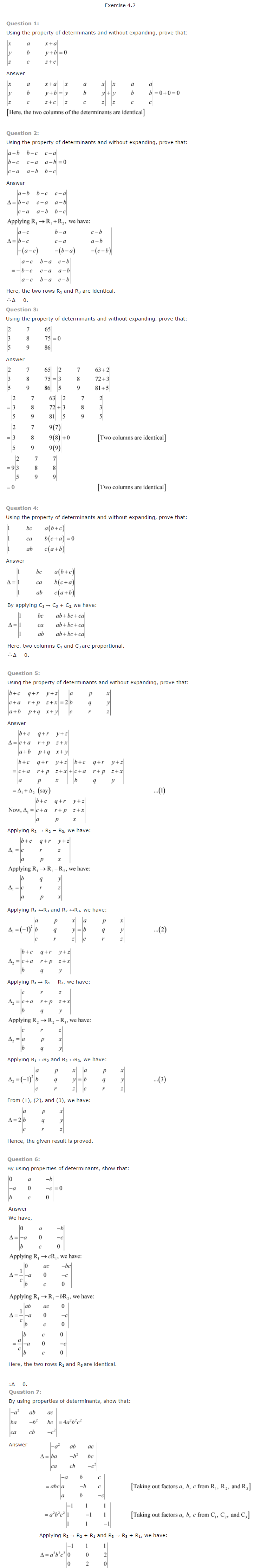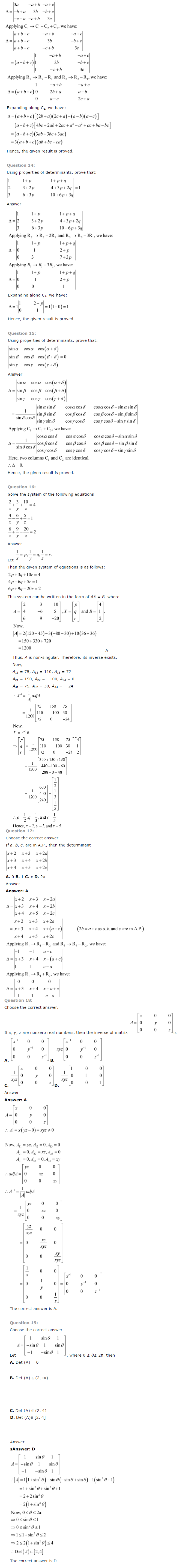# NCERT Solutions Class 12 Maths Ch 4 Determinants

The NCERT Solutions Class 12 Maths Ch 4 Determinants will help in preparation of Class 12 board exams, achieving rank in JEE (Main and Advanced) and scoring marks in other exams. The NCERT Solutions Class 12 Maths Ch 4 Determinants has Ex 4.1 Introduction, Ex 4.2 Determinant, Ex 4.3 Properties of Determinants, Ex 4.4 Area of a Triangle, Ex 4.5 Adjoint and Inverse of a Matrix, Ex 4.6 Applications of Determinants and Matrices, Ex 4.7 Summary.

#### Topics and Exercises in NCERT Solution Class 12 Maths Chapter 4 Determinants:

• Ch 4 Determinants
• Ex 4.1 Introduction
• Ex 4.2 Determinant
• Ex 4.3 Properties of Determinants
• Ex 4.4 Area of a Triangle
• Ex 4.5 Adjoint and Inverse of a Matrix
• Ex 4.6 Applications of Determinants and Matrices
• Ex 4.7 Summary